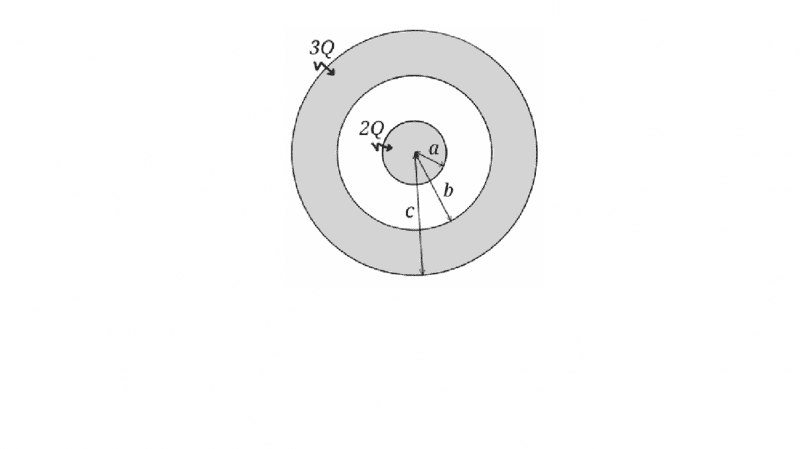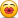# Gauss' Law: Charge distribution on concentric spherical surfaces

Gold Member

## Homework Statement

A metallic sphere of radius a is placed concentrically with a metallic spherical shell with inner radius b and outer radius c. The sphere has a total charge of 2Q and the shell has a total charge of 3Q.
(a) What is the charge distribution? Specifically, what is the charge density in the interior of the metals and at each surface (at r = a, b and c)?
(b) Use Gauss’ law to ﬁnd the electric ﬁeld at every point.Gaussian Law

## The Attempt at a Solution

[/B]Part (a) The charge density of the interior of A is ##ρ=\frac {3(2Q)} {4πa^3}## and for other sphere
##ρ=\frac {3(3Q)} {4π(c^3-b^3}##
The surfaces charge density should be zero.I think like that cause.Electrons cannot pass air.
Electric field will be zero inside the small sphere ##(E=0 , r<a)## then its ##E=\frac {2Q} {4πr^2}## , ##a<r<b## then again zero inside the outer sphere ##(E=0, b<r<c)## then its ##E=\frac {5Q} {4πr^2}## , ##c<r##

Is this true ?

## Answers and Replies

BvU
Science Advisor
Homework Helper
Nope. Charge in conductors is totally mobile. If the charge carries get a chance, they will sit as far away from each other as possible until they can't go any further. (Mathematically: ##\Delta V = 0## -- or rather ##\vec \nabla V = 0## -- in a conductor).

You only show answers, not your work; please show the steps and comment so we can find out whether
Is this true ?
holds ...

Gold Member
If my answers are wrong my logic ahould be wrong too...The problem is Does the -2Q charge will cover the surface of inner sphere.I assumed no cause there no metal betweeen those two charges.But If they can still move then things will change for sure.

ehild
Homework Helper
-2Q is the amount of excess charge on the metal sphere, not two pieces of charge. Charge is not an object, it is attribute of objects. Just like an object have mass, it can have charge. If you charge a metal sphere for -2 C, it means lot and lot charge carriers, electrons. One electron has charge of -1.6˙ 10-19 C, so -2 C means 1.25˙10 19 electrons.˙

Gold Member
-2Q is the amount of excess charge on the metal sphere, not two pieces of charge. Charge is not an object, it is attribute of objects. Just like an object have mass, it can have charge. If you charge a metal sphere for -2 C, it means lot and lot charge carriers, electrons. One electron has charge of -1.6 10-19 C, so -2 C means 1.25 10 19 electrons.
Sure but those -2Q charges will cover the surface ? I mean when I am trying to calculate the surface charge density I ll use that -2Q quantitiy

ehild
Homework Helper
Sure but those -2Q charges will cover the surface ? I mean when I am trying to calculate the surface charge density I ll use that -2Q quantitiy
Yes, they cover the surface. Electrons move free on the metal surfaces, so one electron can be anywhere there. You can calculate the surface charge density by dividing the charge with the surface area.

Gold Member
"what is the charge density in the interior of the metals and at each surface " Then the charge density is 0. but surface charge density is ##E=\frac {-2Q} {4πr^2}## ?

BvU
Science Advisor
Homework Helper
Yes. Don't use the symbol ##E## (it is generally used for the electric field strength; the symbol ##\sigma## is most often used for surface charge density.) And make a habit of mentioning the units too.

Gold Member
Yeah my mistake sorry

BvU
Science Advisor
Homework Helper
Note that there are three surfacess for which you are asked to give the charge density...

Gold Member
So the outer shell inner surgace will be +2Q and outer surface +Q ?

BvU
Science Advisor
Homework Helper
Spot on ! Bingo !However: that's the charges, not the charge densities but I suppose you understand what goes on.

•Arman777
Gold Member
I think I understand the idea...Electric field will be zero every point inside the outer shell till the outer surface then its 3Qk/r^2 ?

Gold Member
Yeah I understand Just have to divide surface areas.And outher shell surface charge density will be 3Q/(Volume of outer shell)

BvU
Science Advisor
Homework Helper
Oops, wrong dimension. You want something in C/m2 !

Gold Member
There should be no surface...

Gold Member
I ll write proparly..everything

Gold Member
Lets call the spere inside A and outside sphere B.The charge density of A, ##ρ=0## (Total charge is zero)
Outer Surface charge density of A ##σ=\frac {-2Q} {4πa^2} \frac{C} {m^2}##
İnner surface charge density of B ##σ=\frac {2Q} {4πb^2} \frac{C} {m^2}##
Charge density of B ##ρ=\frac {3(3Q)} {4π(c^3-b^3)} \frac{C} {m^3}##
Outer surface charge density of B ##σ=\frac {Q} {4πc^2} \frac{C} {m^2}##

Electric Field will change like;
##E=0## till outer surface of B.(till r=c) then it will be ##E=\frac {3Q} {4πε_0r^2} \frac{N} {C}##

Electric field should be zero inside the shell B.

BvU
Science Advisor
Homework Helper
Not good: check total charge on B

Gold Member
İts 3Q

BvU
Science Advisor
Homework Helper
Check again: integrate over the surfaces, integrate over the volume and add up: 6Q !
Gotta run now: concert.

Gold Member
Theres total 5Q charge,how could it be 6Q ?

Gold Member
Check again: integrate over the surfaces, integrate over the volume and add up: 6Q !
Gotta run now: concert.

Oh I understand it was +3Q -2Q passed other surface it become 5Q and one of them is in the outer I think its 5Q ?

Gold Member
Clearly I didnt understand the total idea..I should look more

ehild
Homework Helper
Oh I understand it was +3Q -2Q passed other surface it become 5Q and one of them is in the outer I think its 5Q ?
You are right.
There was some confusion about the sign of charges. The problem text said that "The sphere has a total charge of 2Q and the shell has a total charge of 3Q."
The charge locates on the surfaces. So there is 2Q charge on the surface of the inner sphere of radius a. By induction, there is -2Q charge on the inner surface of the shell, a sphere of radius b, and to make the total charge of the shell 3Q, there must be 5Q charge on the outer surface of radius c.